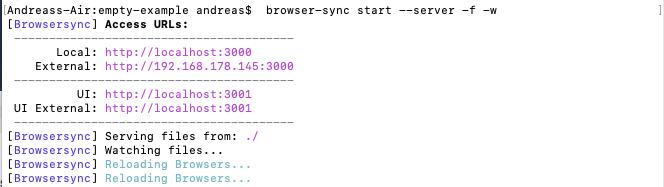# 1. Basics and visuals

### Content

1. Setup
2. Structure of a p5-Sketch
3. A first sketch
4. Basic functions
5. HTML/CSS Framework/implementing p5.js
6. node.js as a framework for testing
7. Drawing sketch on mobile devices
8. More basic drawing
9. Variables
10. Looping / recursion
11. Branching
12. Functions

### 1. Setup

Editor: Sublime Text

### 5. HTML/CSS Framework/implementing p5.js

HTML to host p5.js:

``````
``````

CSS to center canvas

``````
html, body {
height: 100%;
}

body {
margin: 0;
display: flex;

/* This centers our sketch horizontally. */
justify-content: center;

/* This centers our sketch vertically. */
align-items: center;
}
``````

Canvas with full window-size:

``````function setup() {
createCanvas(windowWidth, windowHeight);
background(255, 0, 200);
}

function windowResized() {
resizeCanvas(windowWidth, windowHeight);
}
``````

Place canvas-element in a div-container:

``````function setup() {
var canvas = createCanvas(640, 480);

// Move the canvas into < div id="sketch-container" >.
canvas.parent('sketch-container');

background(100, 100, 100);
}

``````

### 6. node.js as a framework for testing

In terminal:

``npm install -g browser-sync``

Or, if there are rights issues:

``sudo npm install -g browser-sync``

In project folder:

``browser-sync start --server -f -w``

Now we can access our local webpage containing p5 from external devices as tablets or mobile phones. Therefore we use the IP address that Browsersync provides us with:### 7. Drawing sketch on mobile devices

``````function setup() {
createCanvas(640, 480);
background(100);
}

function draw() {
if (mouseIsPressed) {
fill(0);
} else {
fill(255);
}
ellipse(mouseX, mouseY, 80, 80);
}``````

### 8. More basic drawing

ellipse, rect, rectMode, stroke, strokeWeight, fill, color, colorMode, text, textFont, textAlign, loadImage

``````function setup() {
createCanvas(640,480);
background(255);
}

function draw() {
//background(255);
stroke(0,30);
strokeWeight(0.5);
line(300,200,random(640),random(480));
}``````
``````function setup() {
createCanvas(windowHeight, windowWidth);
background(200);
}

function draw() {

if(mouseIsPressed){
if(mouseButton == LEFT){
strokeWeight(random (1,10));
line(pmouseX, pmouseY, mouseX, mouseY);
}

if(mouseButton == RIGHT){
background(200);
}
}
}``````

### 10. Looping / recursion

``````var amount;

function setup() {
amount = 1000;
createCanvas(400, 400);
background(220);
noFill();
}

function draw() {
amount = amount + 10;
background(220);
for (var i = 0; i < 400; i = i+20){
ellipse(200, 200, i, random(10)/random(1));
//ellipse(i, random(20)+200, 3, 3);
//ellipse(random(400), random(400), 10,10);
}
}``````

### 12. Randomness

random()

``````

``````

Perlin Noise

``````let xoff = 0.0;
let yoff = 3;
let nx, ny, lastx, lasty;

function setup(){
createCanvas(600,400);
nx = noise(xoff) * width;
ny = noise(yoff) * height;
lastx = nx;
lasty = ny;
}

function draw() {
//background(204);
xoff = xoff + 0.01;
yoff = yoff + 0.1;

nx = noise(xoff) * width;
ny = noise(yoff) * height;
line(lastx, lasty, nx, ny);
ellipse(nx, ny, 3, 3);
lastx = nx;
lasty = ny;
}``````

### 13. Functions

simple function

``````

``````

simple function with return value

``````

``````

``````var grimesPic;
var step = 15;

}

function setup() {
createCanvas(690, 400);
//image(grimesPic, 0,0, width, height);
grimesPic.resize(width,0);
strokeWeight(4);
noStroke();
fill(0,60);
for (let y = 0; y < height; y += 4) {
for (let x = 0; x < width; x += 12){
var c = grimesPic.get(x,y);
fill(red(c),green(c), blue(c), 40);
stroke(red(c),green(c), blue(c), 40);
ellipse(x,y, 5,5);
line(x,y, x+random(-50,150), y+random(-50,150));
}
}
}

function draw() {

}

``````